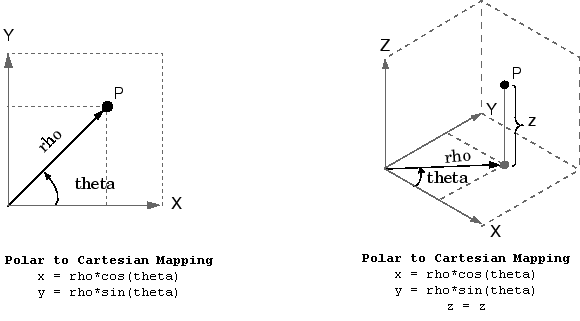Documentation

This is machine translation

Mouseover text to see original. Click the button below to return to the English version of the page.

pol2cart

Transform polar or cylindrical coordinates to Cartesian

Syntax

``````[x,y] = pol2cart(theta,rho)``````
``````[x,y,z] = pol2cart(theta,rho,z)``````

Description

example

``````[x,y] = pol2cart(theta,rho)``` transforms corresponding elements of the polar coordinate arrays `theta` and `rho` to two-dimensional Cartesian, or xy, coordinates.```

example

``````[x,y,z] = pol2cart(theta,rho,z)``` transforms corresponding elements of the cylindrical coordinate arrays `theta`, `rho`, and `z` to three-dimensional Cartesian, or xyz, coordinates.```

Examples

collapse all

Convert the polar coordinates defined by corresponding entries in the matrices `theta` and `rho` to two-dimensional Cartesian coordinates `x` and `y`.

`theta = [0 pi/4 pi/2 pi]`
```theta = 1×4 0 0.7854 1.5708 3.1416 ```
`rho = [5 5 10 10]`
```rho = 1×4 5 5 10 10 ```
`[x,y] = pol2cart(theta,rho)`
```x = 1×4 5.0000 3.5355 0.0000 -10.0000 ```
```y = 1×4 0 3.5355 10.0000 0.0000 ```

Convert the cylindrical coordinates defined by corresponding entries in the matrices `theta`, `rho`, and `z` to three-dimensional Cartesian coordinates `x`, `y`, and `z`.

`theta = [0 pi/4 pi/2 pi]'`
```theta = 4×1 0 0.7854 1.5708 3.1416 ```
`rho = [1 3 4 5]'`
```rho = 4×1 1 3 4 5 ```
`z = [7 8 9 10]'`
```z = 4×1 7 8 9 10 ```
`[x,y,z] = pol2cart(theta,rho,z)`
```x = 4×1 1.0000 2.1213 0.0000 -5.0000 ```
```y = 4×1 0 2.1213 4.0000 0.0000 ```
```z = 4×1 7 8 9 10 ```

Input Arguments

collapse all

Angular coordinate, specified as a scalar, vector, matrix, or multidimensional array. `theta`, `rho`, and `z` must be the same size, or any of them can be scalar.

`theta` is the counterclockwise angle in the x-y plane measured in radians from the positive x-axis.

Data Types: `single` | `double`
Complex Number Support: Yes

Radial coordinate, specified as a scalar, vector, matrix, or multidimensional array. `theta`, `rho`, and `z` must be the same size, or any of them can be scalar.

`rho` is the distance from the origin to a point in the x-y plane.

Data Types: `single` | `double`
Complex Number Support: Yes

Elevation coordinate, specified as a scalar, vector, matrix, or multidimensional array. `theta`, `rho`, and `z` must be the same size, or any of them can be scalar.

`z` is the height above the x-y plane.

Data Types: `single` | `double`
Complex Number Support: Yes

Output Arguments

collapse all

Cartesian coordinates, returned as arrays.

Algorithms

The mapping from polar and cylindrical coordinates to Cartesian coordinates is: